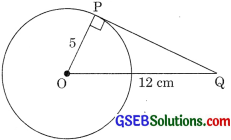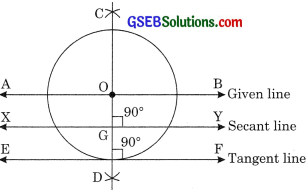# GSEB Solutions Class 10 Maths Chapter 10 Circles Ex 10.1

Gujarat Board GSEB Solutions Class 10 Maths Chapter 10 Circles Ex 10.1 Textbook Questions and Answers.

## Gujarat Board Textbook Solutions Class 10 Maths Chapter 10 Circles Ex 10.1

Question 1.
How many tangents can a circle have?
Solution:
Infinite many tangents. As there are infinitely many points on a circle and all the points can have its tangent.

Question 2.
Fill in the blanks:

1. A tangent to a circle intersects it in …………. point(s).
2. A line intersecting a circle in two points is called a ……….
3. A circle may have ……….. parallel tangents at the most.
4. The common point of a tangent to a circle and the circle is called ……….

Solution:

1. one
2. secant
3. two
4. point of contactQuestion 3.
A tangent PQ at a point P of a circle of radius 5 cm meets a line through the center O at a point Q so that OQ = 12 cm. Length PQ is
(a) 12 cm
(b) 13cm
(c) 8.5 cm
(d) 19 cm
Solution:
(d)From figure,
∠OPQ = 900 (Theorem 10.1)
∴ ΔOPQ is a right-angled triangle.
Using Pythagoras theorem,
OP2 + PQ2 = OQ2
52 + PQ2 = 122
PQ2 = 144 – 25 = 119
∴ PQ = $$\sqrt{119}$$ cmQuestion 4.
Draw a circle and two lines parallel to a given line such that one is tangent and other, a secant to the circle.
Solution:
1. Draw a circle with center O.
2. Draw a line AB passing through O.
3. Draw a perpendicular bisector CD of AB.
4. From D, draw ∠ODF = 90°.
5. Take a point G on OD and draw ∠OGY = 90°.
∴ XY is a secant and EF is a tangent and AB || XY || EF.